# GRE Quantitative Comparison Tip #5 – Estimation with a Twist

Here's the whole series of QC tips:

Tip #1: Dealing with Variables

Tip #2: Striving for Equality

Tip #3: Logic over Algebra

Tip #4: Comparing in Parts

Tip #5: Estimation with a Twist

In my last post, we solved the following question:A. The quantity in Column A is greater

B. The quantity in Column B is greater

C. The two quantities are equal

D. The relationship cannot be determined from the information given

Our approach was to first recognize that two of fractions are approximately \dfrac{1}{2} and two are approximately \dfrac{1}{3}.

Now if we had used these approximations, we would have been left with:This would have made us conclude (incorrectly) that the answer is C.

To solve this question using approximation, we applied a twist. We recognized that \dfrac{213}{428} is a little bit less than \dfrac{1}{2}, which we denoted as \bigg(\dfrac{1}{2}\bigg)^{-}. We also noticed that \dfrac{3007}{9101} is a little bit less than \dfrac{1}{3}, which we denoted as \bigg(\dfrac{1}{3}\bigg)^{-}.

And so on.

With these little twists, we were able to simplify the two columns as:From here, it was clear that the correct answer is B

Okay, now let's see if you can apply this approximation with a twist to solve the following question: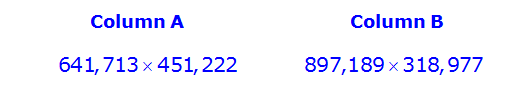Aside: before you read my solutions, see if you can find additional ways to solve this question.

Okay, first we'll solve the question using approximation with a twist, and then we'll solve it using different approaches.

## Approximation with a twist:

First, let's approximate as follows: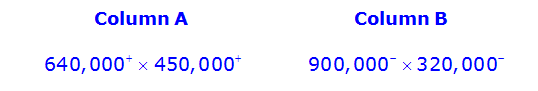From here, we can drop 4 zeroes from each number to get: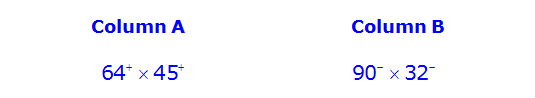At this point, I'll apply a nice rule that says: A \times B = 2A \times {\dfrac{1}{2}}B

In other words, the product of two numbers is equal to the product of twice one number and half the other value.

So, in Column A, we'll double 45^{+} and halve 64^{+} to get:From here, when we compare the products in parts, we can see that Column A must be greater than Column B, so the answer is A.

## Alternative approach #1

As you might have guessed, the two original products are too large to work on a calculator. For example, (641,713) \times (451,222)=289,555,023,286 and this number is too large for the GRE's onscreen calculator. As such, the calculator would display an error message if you tried to perform this calculation.

There are, however, some ways to work around this constraint and still use the calculator.

For example, you could divide each number by 1000 to get: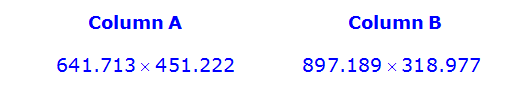this point, the products will still fit into the display of the onscreen calculator and you would clearly see that Column A is greater.

## Alternative approach #2

Another possible approach is to perform the same steps we performed earlier, but stop when we get to: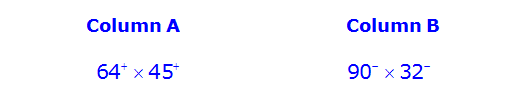Originally, we applied that handy rule where we double one number and halve the other. However, we could also use the calculator at this point.

(64) \times (45)=2880 and (90) \times (32)=2880. This means that (64^{+}) \times (45^{+})=2880^{+} and (90^{-}) \times (32^{-})=2880^{-}.

So, we get: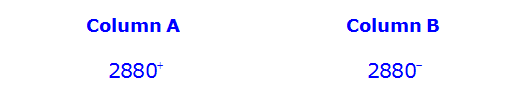Once again, we can see that the answer is A.